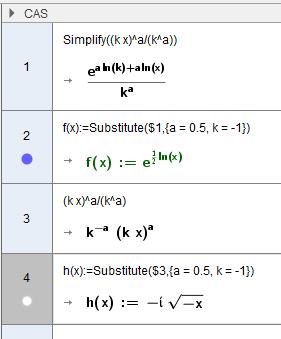# Handling expressions with powers

Jens Akhøj Nielsen shared this problem 5 years ago
Not a Problem

I CAS, the expression (k*x)^a/k^a yeilds k^-a (k*x)^a

Not very impressive! And there are lots of other similar annoying ways, for example the use of rewriting powers as roots.

Regards

jens Akhoej1

worst: Simplify((k*x)^a/(k^a))----> ℯ^((a * log(k)) + (a * log(x))) / k^(a)1

There are plenty of commands to give different forms, eg PartialFractions, Expand, Factor, TrigSimplify, TrigCombine, TrigExpand, Simplify. If one of those doesn't give what you want, please provide an example.

You can also use the "Keep Input" tool.1

I hoped Simplify((k*x)^a/(k^a))--->x^a like Simplify(k^a*x^a)/(k^a)

• Simplify(expand((k*x)^a)/(k^a))
• Expand((k*x)^a)
• Factors((k*x)^a)
• and keep input do not work in this sense

why Simplify((k*x)^a/(k^a))!=Simplify(k^a*x^a)/(k^a)?1

1. (k x)^a/(k^a)

doesn't simplify in general, eg k=-1, a=0.51

no comments. f(x) has a graph